GCSE Maths Ratio and Proportion

Ratio and Proportion

# Ratio And Proportion – Maths GCSE

Here we will learn about ratio and proportion, including forming and sharing with ratios, using solving problems involving direct or inverse proportion, solving problems involving compound measures and finding rates of change.

There are also ratio and proportion worksheets based on Edexcel, AQA and OCR exam questions, along with further guidance on where to go next if you’re still stuck.

## What is ratio and proportion?

Ratio and proportion is an area of mathematics which deals with the relationship between two or more quantities. The understanding of ratio and proportion is a vital part of GCSE mathematics and it links to many of the number, algebra, geometry, probability and statistics topics.

In this page we will link to lots of other pages that will help with a variety of ratio and proportion topics that are examined in both foundation and higher GCSE mathematics. Some have already been seen in key stages 2 and 3.

Each of the sections will include exam practice questions and mark schemes.

### What is ratio and proportion?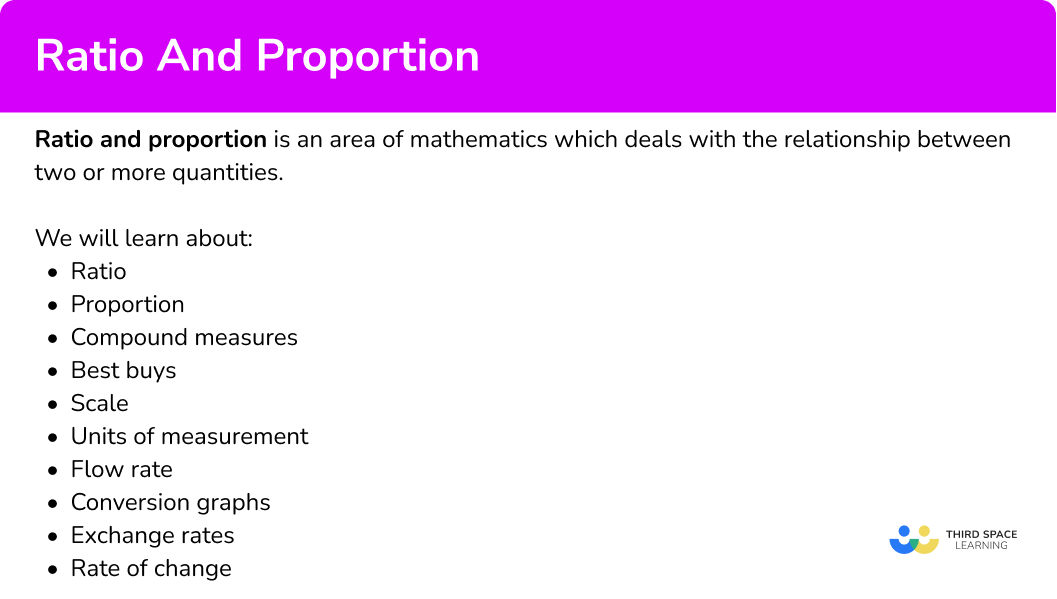### Ratio

Ratio is a relationship between two or more quantities showing the number of times one is contained within the other(s).

Ratios are written in the form a:b, which is said “ a to b ” where a and b are normally integers, fractions or decimals.

They can also be represented in the form \frac{a}{b} (a fraction).

The order of the quantities in the ratio is important.

For example, there are 10 boys in a class and 15 girls. The ratio of boys to girls is 10:15 which we say as “ 10 to 15 ”.

We can use ratios in a variety of ways,

• Working out ratio
• Simplifying ratios
• Dividing (sharing) in ratios
• Ratios to fractions
• Ratio problem solving
• Ratio scales

Step-by-step guide: Ratio

### Proportion

Proportion is a type of relationship between two variables linked by a constant

There are two types of proportion, direct proportion and inverse proportion. They can also be referred to as direct variation and inverse variation

Equations can be formed to represent proportional relationships.

The symbol ‘\propto’ means ‘is proportional to’.

If y is directly proportional to x we can write it as y\propto{x}.

We can then rewrite this using a constant of proportionality usually denoted by the letter ‘k’.

The direct proportion formula is y=kx.

If y is inversely proportional to x we can write it as y\propto\frac{1}{x} .

We can then rewrite this using a constant of proportionality usually denoted by the letter ‘k’.

The inverse proportion formula is y=\frac{k}{x}.

We can also have proportional relationships involving powers with equations such as y=k{x}^{n}, \ y=k\sqrt[n]{x}, \ y=\frac{k}{{x}^{n}} and y=\frac{k}{\sqrt[n]{x}}.

Step-by-step guide: Proportion

## Compound measures

Compound measures are measures which are found from two other measures.

The most common compound measures studied at GCSE Maths are speed, density and pressure.

There are other compound measures such as rates of pay (for example, pounds (£) per hour) or flow rate (for example, litres per minute). We can also use compound measures when calculating a best buy (for example, grams per pence).

Step-by-step guide: Compound measures (coming soon)

• Speed

Speed is a compound measure made from dividing a unit of distance by a unit of time. Speed is a measure of how much an object has travelled over a certain time period. The different units for speed include metres per second (m/s) and kilometres per hour (km/h).

The speed formula is,

\text{Speed}=\frac{\text{distance}}{\text{time}}.

Step-by-step guide: Speed distance time

• Density

Density is a compound measure made from dividing a unit of mass by a unit of volume. Density is a measure of how the amount of matter there is in a certain volume. The different units for density include grams per cubic centimetre (g/cm^{3}) and kilograms per cubic metre (kg/m^{3}).

The density formula is,

\text{Density}=\frac{\text{mass}}{\text{volume}}.

Step-by-step guide: Mass density volume

• Pressure

Pressure is a compound measure made from dividing a unit of force by a unit of area. Pressure is defined as the force per unit area. The standard unit of pressure is Pascals (Pa). One Pascal is equal to 1 \ N/m^{2}. We need to know that pressure is calculated by dividing the amount of force by the area.

The pressure formula is,

\text{Pressure}=\frac{\text{force}}{\text{area}}.

Step-by-step guide: Pressure force area

Best buy problems involve assessing which item is the best value for money.

To do this we we could use the following methods,

Unitary method

This involves calculating the price per unit for each item.

Common multiples

Here we find a common multiple of the number of units we have and use this to make comparisons.

Shop deals

We interpret deals such as ‘3 for 2’, ‘buy one get one free’ or a percentage extra free.

Exchange rates

If the prices are in different currencies, we convert them to the same currency to compare them.

## Scale maths

Scale maths is a way of enlarging an object.

If we have two shapes that are similar, one will be a scale diagram of the other. Usually we need to find the scale factor of enlargement or find a missing length, area or volume.

Scale maths is used for real world scale drawings as it is much easier to create a scale drawing of an object, than to draw the object using actual distances

The ratio usually takes the form 1:n of the model/plan to the actual distance.

For example, below is a scale diagram of a garden where 1 square is equal to 0.5 metres.

Writing this as a ratio we get \bf{1} square: \bf{0.5 \ m} .

Step-by-step guide: Scale maths

## Units of measurement

Units of measurement are used to measure different quantities.

The main metric units of measurement are here,

We also need to know how to convert between different units of measurement.

We also need to know about imperial units of measurement and units of measurement for area and volume.

Step-by-step guide: Units of measurement

## Flow rate

Flow rate is the term used to describe the rate at which a fluid or substance flows into or out of an object.

To calculate flow rate we can use information about the change in capacity of the substance and the amount of time taken for that change to occur.

\text{Flow rate}=\frac{\text{change in volume}}{\text{time taken}}

For example, a tap is used to fill a container in the shape of a cuboid measuring 1.5 \ m by 2 \ m by 0.4 \ m.

The tap releases water at a flow rate of 5 litres per minute.

Find the time taken for the container to be filled.

Step-by-step guide: Flow rate

## Conversion graphs

Conversion graphs are straight line graphs that show a relationship between two units and can be used to convert from one to another. They are very useful to solve real-life problems.

Some conversion graphs can show a direct proportion between two units, for example, converting between two currencies to show an exchange rate, such as Pounds Sterling to US Dollars.

Step-by-step guide: Conversion graphs

## Exchange rates

An exchange rate is the rate at which the money of one country can be exchanged for the money of another country. Using the exchange rate we can convert between two currencies.

To convert from British pounds to a foreign currency you must multiply the currency in pounds by the exchange rate.

For example, £1 is equivalent to \$1.87 \ AUD and so for any amount given in British pounds, we multiply that value by 1.87 to get the number of Australian dollars. To convert from a foreign currency into British pounds you must divide by the exchange rate. For example, to convert Australian dollars back to British pounds, we divide the number of Australian dollars by 1.87. Step-by-step guide: Exchange rates ## Rate of change Rates of change relate to what degree one variable changes in relation to another. A rate of change can be calculated by finding the gradient of a straight line, either at a point or between two points. For example, below is a straight line graph. The gradient of the line is m=\frac{\text{change in }y}{\text{change in }x}=\frac{3}{2}=1.5. Sometimes we need to find the instantaneous rate of change. This is the rate of change at a specific point. It is found by calculating the gradient of a curve/line at a particular point using a tangent line. A tangent line is a line that just touches a curve at a single point, it matches the curve’s gradient at that point. We may also need to find the average rate of change from a curve. This is the rate of change between two points where the rate of change is not constant. It is found by calculating the gradient of a chord between two points. Step-by-step guide: Rate of change ### Common misconceptions • Ratio written in the wrong order A common error is to write the parts of the ratio in the wrong order. For example, the number of dogs to cats is given as the ratio 12:13 but the solution is incorrectly written as 13:12. • Ratios and fractions confusion For example, the ratio 2:3 is incorrectly expressed as the fraction \frac{2}{3} and rather than the correct answer of \frac{2}{5}. This is a misunderstanding of the sum of the parts of the ratio. The sum of all of the parts of the ratio gives us the denominator of the fraction. • Incorrect use of the exchange rate when converting currencies If £1= \$1.30, to convert £ to \$we need to multiply by 1.30. To convert \$ to £ we need to divide by 1.30, not the other way around.

• Converting units

Sometimes the units need to be converted, so it is important to be able to confidently convert between different metric units.

For example, a map scale is given as 1:25000 which means that 1 \ cm on the map is equivalent to 25000 \ cm in real life. If the answer needs to be written in kilometres, the real life value in centimetres must be divided by 100 \ 000 to get the same measurement in kilometres. 1:25 \ 000 = 1 \ cm: 0.25 \ km.

### Practice ratio and proportion questions

1. The fraction of bananas in a bowl is \frac{8}{15}. Calculate the ratio of bananas to other pieces of fruit in the bowl.

8:158:78:237:8Let the total number of pieces of fruit be 15. The number of bananas is 8. The number of other pieces of fruit is therefore 7. The ratio of bananas to other pieces of fruit is therefore 8:7.

2. If it takes 5 workers 12 hours to do a job, how long would it take 9 workers to complete the same job? Give your answer in hours and minutes.

3 hours 45 minutes21 hours 6 minutes7 hours 6 minutes6 hours 40 minutesThis is an inverse proportion problem.

The total time needed to complete the job is

k=5\times 12=60 hours.

This means that y=\frac{60}{x} where y is the number of hours it takes x people to complete a job.

Sharing this time between 9 workers would be

y=60\div 9=6\frac{2}{3}.

Two thirds of an hour ( 60 minutes) is calculated by multiplying 60 by two thirds.

60\times\frac{2}{3}=40 minutes

The final answer would be 6 hours 40 minutes.

3. Which of these graphs indicates that y\propto\frac{1}{x}?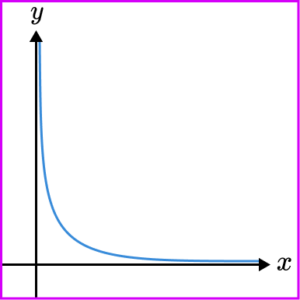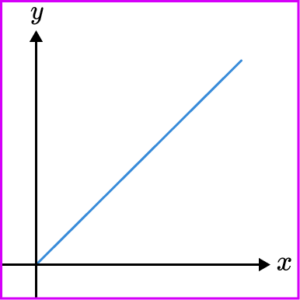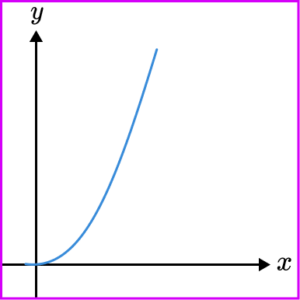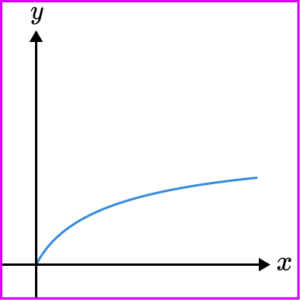y\propto\frac{1}{x} becomes y=\frac{k}{x} and so a reciprocal graph is required. The graph must be a smooth curve, and does not touch either axis.

4. Find the density of an object when the mass of the object is 350 \ g and its volume is 25 \ cm^{3}.

325 \ g/cm^3375 \ g/cm^314 \ g/cm^37 \ g/cm^3\text{Density}=\frac{\text{mass}}{\text{volume}}=\frac{350}{25}=14 \ g/cm^3

5. A hose pipe is used to fill a water butt. Water flows at a rate of 3.5 litres per minute and fills the water butt in 12 minutes and 52 seconds. Calculate the volume of the water butt to the nearest litre.

51 litres44 litres45 litres42 litresConvert the time into the decimalised form, then use the formula

\text{Flow rate}=\frac{\text{change in volume}}{\text{time taken}}.

By multiplying both sides of the formula by the time taken, we can change the subject to be the volume.

\text{Volume}=\text{flow rate}\times \text{time taken}.

Substituting the values for the flow rate and the time into the formula, we have

3.5\times{12}\frac{52}{60}=45.0\dot{3}.

The volume will be 45 litres, to the nearest litre.

6. Lucy is buying some items from an online shop which will accept payments in pounds and euros.

She buys a watch costing £150, a pendant costing £280 and a frame costing £17.

The exchange rate is £1 = €1.27 . Work out the cost of her bill in euros.

€567.69€447€351.97€546.10Adding up the prices first, we have

\pounds 150 + \pounds 280+ \pounds 17=\pounds 447.

Multiplying the total by the exchange rate 1.27, we have

447\times 1.27=567.69.

This gives €567.69.

### Ratio and proportion GCSE questions

1. Imogen and Samuel share some sweets in the ratio of 7:5.

Imogen has 12 more sweets than Samuel. How many sweets were there altogether

(3 marks)

2 shares is equivalent to 12 sweets or 1 share is equivalent to 6 sweets.

(1)

42:30

(1)

72 sweets

(1)

2. Stones of different mass M \ kg are dropped into sand and make a circular crater of diameter D \ cm.

The diameter of the crater is directly proportional to the square root of the mass.

A stone of mass 4 \ kg makes a crater of diameter 6 \ cm.

(a) Write a formula for D in terms of M.

(b) Find the diameter of the crater made by a stone of mass 9 \ kg.

(c) Find the mass of the stone that makes a crater of diameter 12 \ cm.

(7 marks)

(a)

Form an equation with k, \ D=k\sqrt{M} .

(1)

k=\frac{6}{\sqrt{4}}=\frac{6}{2}=3

(1)

D=3\sqrt{M}

(1)

(b)

Substitute M=9 into the equation, D=3\sqrt{9}.

(1)

D = 9 \ cm

(1)

(b)

Substitute D=12 into the equation, 12=3\sqrt{M} .

(1)

16 \ kg

(1)

3. Joseph buys a camera in the USA for \$330. Amelia buys the same camera in the UK for £255. The exchange rate is £1 = \$1.32.

Where is the camera the cheapest, USA or UK?

(2 marks)

255 \times 1.32 or 330 \div 1.32

(1)

USA

(1)

## Still stuck?

Prepare your KS4 students for maths GCSEs success with Third Space Learning. Weekly online one to one GCSE maths revision lessons delivered by expert maths tutors.

Find out more about our GCSE maths tuition programme.